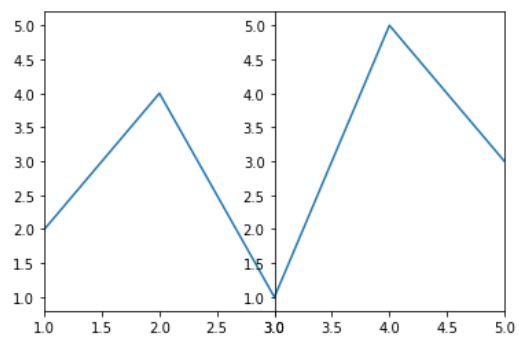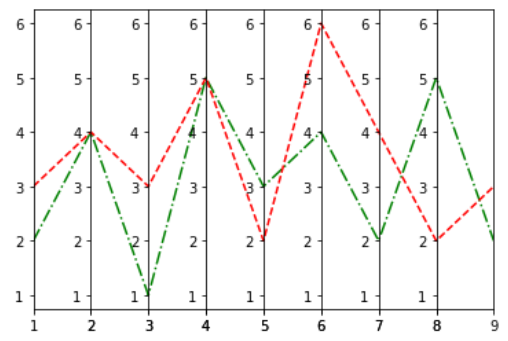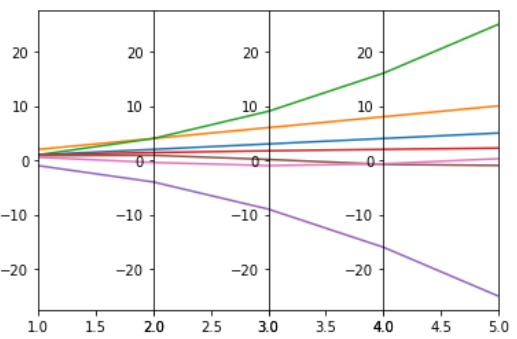Parallel Coordinates in Matplotlib

• Last Updated : 24 Jan, 2021

In this article, we will learn How to Plot Parallel Coordinates in Matplotlib. So, first discuss some concepts :

• Matplotlib may be a tremendous visualization library in Python for 2D plots of arrays. Matplotlib could also be a multi-platform data visualization library built on NumPy arrays and designed to work with the broader SciPy stack. It was introduced by John Hunter within the year 2002.
• One of the best benefits of visualization is that it allows us visual access to large amounts of knowledge in easily digestible visuals. Matplotlib consists of several plots like line, bar, scatter, histogram etc.
• Parallel coordinates may be a method for exploring the spread of multidimensional data on a categorical response, and taking a look at whether there’re any trends to the features.
• Two and three-dimensional data are often viewed relatively straight-forwardly using traditional plot types. Even with four dimensional data, we will often find how to display the info.

Steps Needed

1. Import libraries (matplotlib)
3. Make subplots with share y-axes equal to False
4. Plot all the subplots
5. Set x-axis limit for x-axis labels
6. Make the width space zero
7. Show the plot for final output

Examples

Here, we will discuss some examples by applying above mentioned steps. In these examples, we will work on dummy data in linear and multi-dimensions.

Example 1: (Simple Parallel Coordinate Plot)

Python3

 # import packagesimport matplotlib.pyplot as plt  # create datax=[1,2,3,4,5]y=[2,4,1,5,3]  # make subplotsfig,(ax1,ax2) = plt.subplots(1, 2, sharey=False)  # plot the subplotsax1.plot(x,y)ax2.plot(x,y)  # set x limitsax1.set_xlim([ x,x])ax2.set_xlim([ x,x])  # set width space to zeroplt.subplots_adjust(wspace=0)  # show the plotsplt.show()

Output:Example 2: (Parallel Coordinate Plot with multiple lines and multiple axes)

Python3

 # import packagesimport matplotlib.pyplot as plt  # create datax=[1,2,3,4,5,6,7,8,9]y1=[2,4,1,5,3,4,2,5,2]y2=[3,4,3,5,2,6,4,2,3]  # make subplotsfig, (ax1,ax2,ax3,ax4,ax5,ax6,ax7,ax8) = plt.subplots(1, 8, sharey=False)ax = (ax1,ax2,ax3,ax4,ax5,ax6,ax7,ax8)  # plot subplots and set xlimitfor i in range(8):    ax[i].plot(x,y1,'g-.',x,y2,'r--')    ax[i].set_xlim([ x[i],x[i+1]])  # set width space to zeroplt.subplots_adjust(wspace=0)  # show plotplt.show()

Output :Example 3: (Parallel Coordinate Plot over multi-dimensions data)

Python3

 # import packagesimport numpy as npimport matplotlib.pyplot as plt  # create datax = np.arange(1,6)data = [x,x*2,x*x,np.sqrt(x),-x*x,np.sin(x),np.cos(x)]print(data)  # make subplotsfig, (ax1,ax2,ax3,ax4) = plt.subplots(1, 4, sharey=False)ax = (ax1,ax2,ax3,ax4)  # plot subplots and set xlimitfor i in range(4):    for j in range(len(data)):        ax[i].plot(data,data[j])    ax[i].set_xlim([x[i],x[i+1]])  # set width space to zeroplt.subplots_adjust(wspace=0)  # show plotplt.show()

Output :My Personal Notes arrow_drop_up